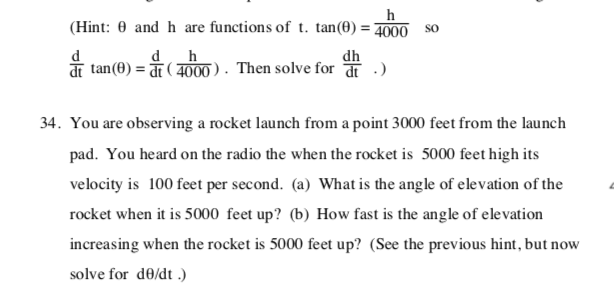# Solved: Functions Of T. Tan(0) = 4000 So (Hint: 0 And H Are D Dh Dt Tan(0) Dt (4000) . Then Solve For Dt 34. You Are Observing A Rocket Launch From A Point 3000 Feet From The Launch Pad. You Heard On

By |functions of t. tan(0) = 4000 so (Hint: 0 and h are d dh dt tan(0) dt (4000) . Then solve for dt 34. You are observing a rocket launch from a point 3000 feet from the launch pad. You heard on the radio the when the rocket is 5000 feet high its velocity is 100 feet per second. (a) What is the angle of elevation of the rocket when it is 5000 feet up? (b) How fast is the angle of elevation increasing when the rocket is 5000 feet up? (See the previous hint, but now solve for de/dt